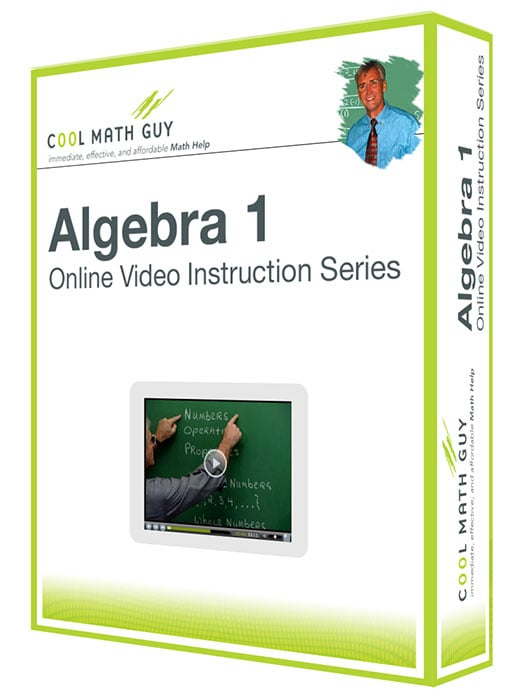Algebra 1 - Educators Rate

Minimum 10 Students

Algebra 1 – Educators Rate
•\$50.00

Core topics include solving linear equations and inequalities, graphing equations and inequalities with some use of the graphing calculator, exponents, polynomials, factoring, rational expressions and equations, systems of linear equations and inequalities, radical expressions and equations, and solving quadratic equations. Algebra I follows Prealgebra in the sequence of math courses and is often used as a developmental course at the college level under the name Elementary Algebra.

Reviews

There are no reviews yet.

Only logged in customers who have purchased this product may leave a review.